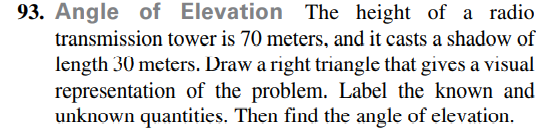### Still have math questions?93. Angle of Elevation The height of a radio transmission tower is $$70$$ meters, and it casts a shadow of length $$30$$ meters. Draw a right triangle that gives a visual representation of the problem. Label the known and unknown quantities. Then find the angle of elevation.